# Sudoku Solver

### OpenCV project for reading and solving Sudokus

#### Category: Projects Tags: Algorithm, OpenCV

Posted by Anant Jain on July 31, 2016

Sudoku Solver is the collection of very basic image processing techniques. A very good way to start is the OpenCV library which can be compiled on almost all the platforms. OpenCV(open source computer vision )is a library of programming functions mainly aimed at real time computer vision. Through this project ,my main motivation was to explore what OpenCV offers in a little bit detail . There are already many blogs dealing with how to recognise a whole sudoku puzzle but it  was nevertheless  a pleasant experience doing it on my own and writing this blog ( It is pretty obvious that I too would have been lost without all those online resources , blogs , and documentations.)

### What actually this project does?

It takes an input image of a sudoku and processes the image and identifies the all the whole suduko and return the answer of the sudoku. It involves two major challenges:

1. Image recognition
2. Solving the sudoku puzzle

I was more interested in the image processing part as there are fixed algorithm for solving  the sudoku puzzle so my main focus in this blog would be the image processing part.

### The major steps this will involve are:

2.  Preprocessing the image ( removal of noises and thresholding the image)
3.  Finding the sudoku square out of the whole image
4.  Extracting the sub-grids of the sudoku.
5. Recognising the digits (OCR)

### We also need to make a few assumptions :

1. In the image , the largest square would be that of the sudoku image
2. The puzzle would be oriented reasonably oriented.

So let’s get started,

First of all we need is an input image.  We need to load a sudoku image .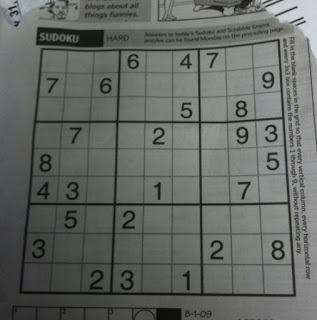After loading the image ,the first thing to do in any image processing problem is to reduce the amount of data you are dealing with.We started with a full colour high resolution image.The first thing we can do is to convert the image into a gray scale as looking at our sample image having colour is of no use to us.

### Image Processing:

After we have converted the image into a gray scale image we need to remove the noises from the image and smoothen the image as without smoothing the image we deal with extra objects which are not needed so it is necessary to remove the noises. There are many functions available in the OpenCV library for blurring the image like blur , GaussianBlur , MedianBlur . I tried them all and the best result I got out of them was with gaussian blur so I used it . Next , what we need to do is to remove other extra information . We are going to threshold the image that is we have either the foreground pixel or the background pixel. There are variety of thresholding techniques available to us in OpenCV library. My personal favourite is a simple adaptive threshold .For each pixel in the image it takes the average value of the surrounding area.

I  have done just noise removal and adaptive thresholding and  it is working so haven’t done anything extra. Below is the result: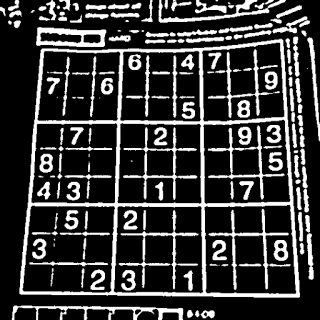### Extracting the Sudoku :

Now after thresholding we need to find out the sudoku square , for this we made an assumption the main thing in our image would be the sudoku so we need to find the square with the largest area and it would be our sudoku.

So one thing is very important the sudoku square should be largest otherwise our method fails.

We start by finding the countours in our thresholded image.

Now we find the blob with maximum area .First we filter them by area . We consider the blob for the next processing only if its area is greater than a particular value (here , it is 50) . Next , we find out the area of each blob and hence extract the blob which has the maximum area.

Now after finding the blob with maximum area we approximate the countour into a polygon. It removes the unwanted coordiante values in the countour and keeps only the corners.

Now we draw the contour on our image just to check it.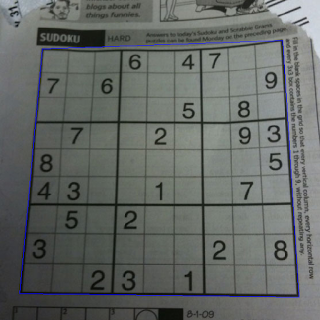Now we have found out the boundary of the sudoku and next we need to to do is extract this much part and then work on it.

As we have approximated the contours into a square or rectangle we will get only four coordinate that are the corners and now what we need to do is find out which coordinate is of the top-left corner , top-right corner , bottom-left corner , bottom-right corner. As the order of contours in all the image will not be fixed we need to find it out as we have to map the top-left to [0,0] for new image and bottom-right to [449,449]  , as we are creating an image of [450,450] you can do it of whichever size you want , therefore we need to identify the correct order of corners otherwise we will get rotated images. The logic I choose to find the respective corners was : First take the sum of x , y coordinates TOP -LEFT has least sum and BOTTOM-RIGHT has the maximum sum. Now the difference  i.e y-x TOP-RIGHT has minimum and BOTTOM-LEFT has maximum sum.

Now we have 4 points in order and now we need corresponding points where they should be mapped.

Now we have the input and output array both what we need to do is apply prespective transformation to get the sudoku part required. Prespective transformation maps a point given by x, y in one quadilateral to a new point X ,Y in another quadilateral.

### The result we get is: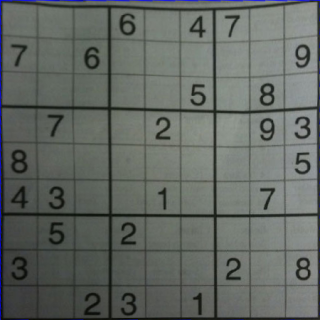Now apply the pre-processing as we did earlier and get the thresholded image and now we need to extract each grids centre the digit and then finally apply OCR. So, we can extract each grids as we know our image is a matrix of 450450 and therefore our every sub grids will be a matrix of 5050 so we can extract each grid by extracting the sub grids of 50*50 and store all the images in a vector.

Now we have each small grid and it may contain digits or not so we will set a threshold pixel if the image contains pixels greater than that then it may contain a digit otherwise it doesnot and now the images with pixels greater than threshold you need to extract the digit and center it rather than testing the image directly as it will increase the changes of correct recogniton. We can extract the digit and centre it in same way as we extracted the main grid , find the  contour  and bound it by rectangle and find the bounding rectangle with greatest area then resize it.(Assumption : the subgrids containing digits will have the digits as the main part).

### The results is as follows: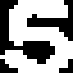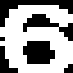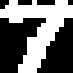Now we have all the digits centered what we now need to do is apply OCR . There are huge number of techniques for implementing OCR and huge number of pattern recognition algorithm and for my implementation i choose K-Nearest Neighbour algorithm as it is already available in OpenCV library.The algorithm caches all training samples and predicts responses for new sample by analyzing a certain number of the nearest neighbour of the sample using voting and calculated mean. For it you need to create sample data and train those images and recognise digits from previously trained data. I created training samples by collecting images from various sudoku .My training data can be found out on this link.

This is the code for training the data :

This trains the data and to recognise the number we use the find_nearest of the KNearest class in OpenCV library.

The accuracy of this method is upto 90% . So to increase the accuracy you may increase number of the training samples but it may not give very good results ,so to improve the accuracy try out other algorithms available which are more accurate.

Although,I did not get 100% correct results but anyways it was overall a great learning experience.

The whole source code can be found here.